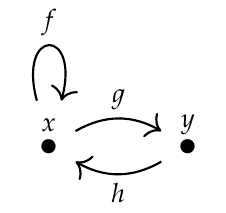Keith wrote:

> In order to do matrix multiplication, we need a multiplication and a sum operator. If we take composition {∘} to be our multiplication, what is the sum? Just simple old set union {∪}?

I intended people to just multiply and add without worrying about what it means! Physicists do this all the time - it's very useful. They call it "formal manipulations". Mathematicians call it "abstract algebra" and can't resist making the rules precise.

In other words: make up something called \$$\cdot\$$ for multiplication, something called \$$+\$$ for addition - and don't ask what they mean, just have them obey the usual rules of a [rig](https://en.wikipedia.org/wiki/Semiring):

* addition and multiplication are associative,

* anything plus 0 is itself,

* anything times 0 is zero,

* anything times 1 is itself.

This will let you start multiplying the matrix

$\left( \begin{array}{cc} f & g \\\\ h & 0 \end{array} \right)$

by itself, over and over... and you'll see all the paths in this graph start to emerge:You'll also see why I didn't want multiplication to be commutative.

Of course you should then wonder what's going on. You might decide that \$$\cdot\$$ means the same thing as composition \$$\circ\$$ in our category. You might reinvent a whole bunch of math. It's more fun than learning it from a book!

Fredrick was doing something like this in the comment right before this one, but he was working less abstractly: he seems to be taking \$$\cdot\$$ to be composition and \$$+\$$ to be union of [multisets](https://en.wikipedia.org/wiki/Multiset), approximately speaking. But for this you need to decide what it means to compose two finite multisets of morphisms: that's where the distributive law comes in!

There's also the usual problem of whether you use \$$f\circ g\$$ to mean "first \$$g\$$ then \$$f\$$" or something like \$$f ;g\$$ to mean "first \$$f\$$ then \$$g\$$". As usual, it doesn't matter much as long as you're consistent. The second convention is a bit less annoying.